Next: Exercises Up: Damped and Driven Harmonic Previous: Driven LCR Circuits

# Transient Oscillator Response

The time evolution of the driven mechanical oscillator discussed in Section 3.4 is governed by the driven damped harmonic oscillator equation,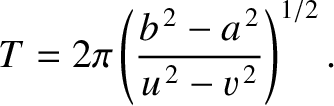(131)

Recall that the steady (i.e., constant amplitude) solution to this equation that we found earlier takes the form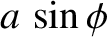(132)

where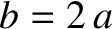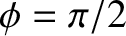(133)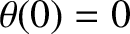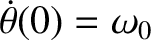(134)

Equation (131) is a second-order ordinary differential equation, which means that its general solution should contain two arbitrary constants (Riley 1974). However, Equation (132) contains no arbitrary constants. It follows that the right-hand side of (132) cannot be the most general solution to the driven damped harmonic oscillator equation, (131). However, if we add any solution of the undriven damped harmonic oscillator equation,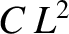(135)

to the right-hand side of (132) then the result will still be a solution to Equation (131). From Section 3.1, the most general solution to the preceding equation can be written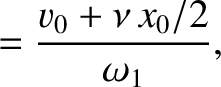(136)

where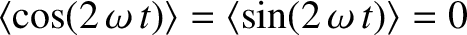, andand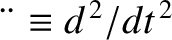are arbitrary constants. [In terms of the standard solution, (72),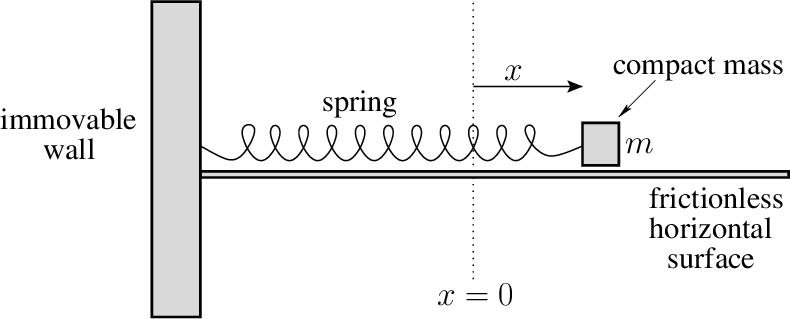and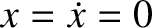.] Thus, a more general solution to Equation (131) is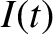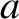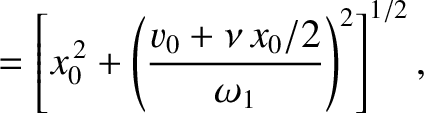(137)

In fact, because the preceding solution contains two arbitrary constants, we can be sure that it is the most general solution. The arbitrary constants,and, are determined by the initial conditions. Thus, the most general solution to the driven damped harmonic oscillator equation, (131), consists of two parts. First, the solution (132), which oscillates at the driving frequency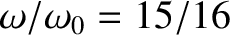with a constant amplitude, and which is independent of the initial conditions. Second, the solution (136), which oscillates at the natural frequency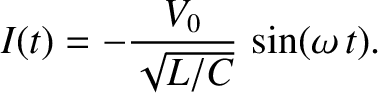with an amplitude that decays exponentially in time, and which depends on the initial conditions. The former is termed the time asymptotic solution, because if we wait long enough then it becomes dominant. The latter is called the transient solution, because if we wait long enough then it decays away.

Suppose, for the sake of argument, that the system is initially in its equilibrium state. In other words,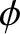. It follows from Equation (137) that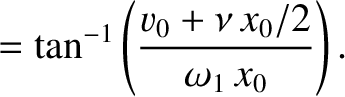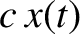(138)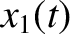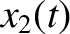(139)

The preceding equations can be solved to give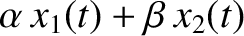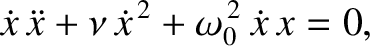(140)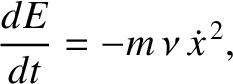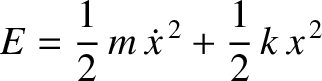(141)

According to the analysis in Section 3.4, for driving frequencies close to the resonant frequency (i.e.,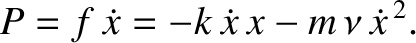), we can write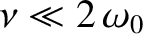(142)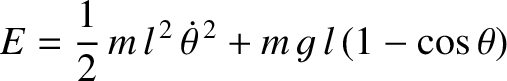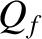(143)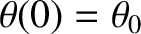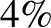(144)

Hence, in this case, the solution (137), combined with Equations (140)-(144), reduces to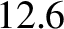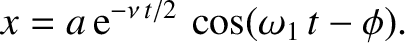(145)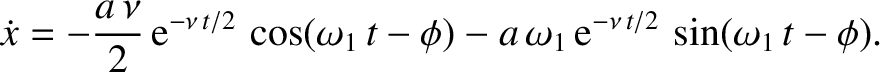There are a number of interesting cases that are worth discussing. Consider, first, the situation in which the driving frequency is equal to the resonant frequency: that is,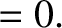. In this case, Equation (145) reduces to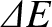(146)

because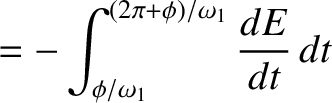. Thus, the driven response oscillates at the resonant frequency,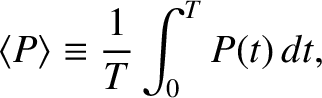, because both the time asymptotic and transient solutions oscillate at this frequency. However, the amplitude of the oscillation grows monotonically as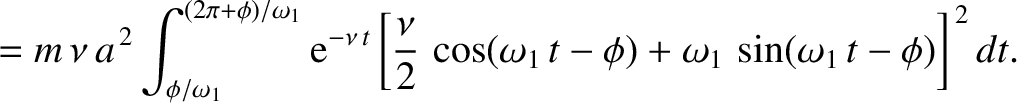, and so takes a time of order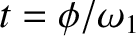to attain its final value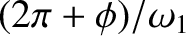, which is, of course, larger that the driving amplitude by the resonant amplification factor (or quality factor),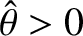. This behavior is illustrated in Figure 12. [Here,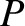and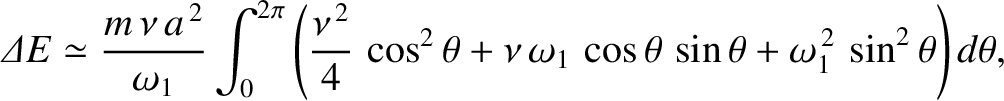. The solid curve shows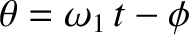, and the dashed curves show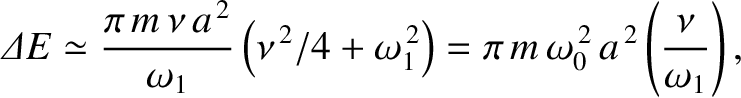.]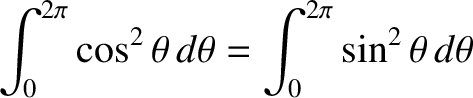Consider the situation in which there is no damping, so that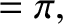. In this case, Equation (145) yields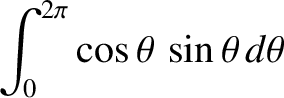(147)

where use has been made of the trigonometry identity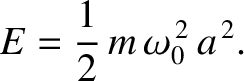. (See Appendix B.) It can be seen that the driven response oscillates relatively rapidly at the sum frequency''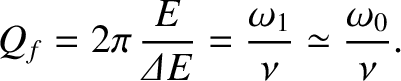with an amplitude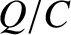that modulates relatively slowly at the difference frequency''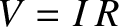. (Recall, that we are assuming thatis close to.) This behavior is illustrated in Figure 13. [Here,and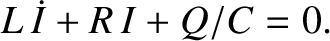. The solid curve shows, and the dashed curves show.] The amplitude modulations shown in Figure 13 are called beats, and are produced whenever two sinusoidal oscillations of similar amplitude, and slightly different frequency, are superposed. In this case, the two oscillations are the time asymptotic solution, which oscillates at the driving frequency,, and the transient solution, which oscillates at the resonant frequency,. The beats modulate at the difference frequency,. In the limit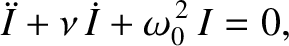, Equation (147) yields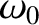(148)

because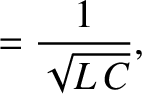when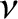. (See Appendix B.) Thus, the resonant response of a driven undamped oscillator is an oscillation at the resonant frequency whose amplitude,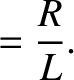, increases linearly in time. In this case, the period of the beats has effectively become infinite.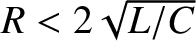Finally, Figure 14 illustrates the non-resonant response of a driven damped harmonic oscillator, obtained from Equation (145). [Here,,, and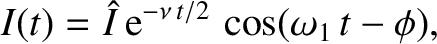.] It can be seen that the driven response grows, showing some initial evidence of beat modulation, but eventually settles down to a steady pattern of oscillation. This behavior occurs because the transient solution, which is needed to produce beats, initially grows, but then damps away, leaving behind the constant amplitude time asymptotic solution.Next: Exercises Up: Damped and Driven Harmonic Previous: Driven LCR Circuits
Richard Fitzpatrick 2013-04-08